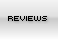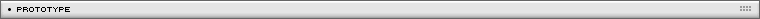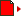A* Pathfinding
[ August 13, 2003 ] by Zeh Fernando
In this example Zeh shows a complete implementation of the A* pathfinding algorithm.

Click on the map to calculate the path starting from the soldier position

Download the source code of this prototype

This function finds the path between two given points using an A* algorithm. It's usually needed on games that need pathfinding/navigating AI.

It uses a bidimensional map (the MAP), a START point (defined as an Y and X value, in this order to maintain standard with the way the map is built), and an END point (Y and X values).
Each MAP cell have a value of 0 (when it means a wall, or a cell it can't be visited on the route) or a value of 1 or greater. In this case, this value means the COST to navigate through that point. On an standard map, for example, a value of 1 would be used for a road, a value of 2 for normal terrain, and a value of 3 for mountains.
You can also modify the "CONSTANT variables" inside the function. For instance, you can set ALLOW_DIAGONAL to to TRUE to allow diagonal movements or to FALSE to disallow it.

AN EXAMPLE OF USAGE

```// Creates a map

map = [[2,2,2,2,2,2,2,2,2,2,1,2,2,2,2],       [2,2,2,2,2,2,2,2,2,2,1,2,2,2,2],       [2,2,2,2,2,2,2,2,2,2,1,2,2,2,2],       [2,2,2,2,2,2,2,2,2,2,1,2,2,2,2],       [2,2,2,2,2,2,2,2,2,2,1,2,2,2,2],       [2,2,2,2,2,2,2,2,2,2,1,2,2,2,2],       [2,2,0,0,0,0,0,0,0,2,1,2,2,2,2],       [2,2,2,2,2,2,2,2,2,2,1,2,2,2,2],       [2,2,2,2,2,2,2,2,2,2,1,2,2,2,2],       [2,2,2,2,2,2,2,2,2,2,1,2,2,2,2],       [2,2,2,2,2,2,2,2,2,2,1,2,2,2,2],       [2,2,2,2,2,2,2,2,2,2,1,2,2,2,2]];

// Finds the path from point 2,2 to point 9,9

fpath = findPath (map, 2, 2, 9, 9);

// Draw results

for (i=0; i<map.length; i++) {   for (j=0; j<map.length; j++) {      switch (map[i][j]) {      case 0:         _root.beginFill (0x333366);         break;      case 2:         _root.beginFill (0xdddddd);         break;      case 1:         _root.beginFill (0xeeeeee);         break;      }      _root.moveTo (j*20, i*20);      _root.lineTo ((j+1)*20,i*20);      _root.lineTo ((j+1)*20,(i+1)*20);      _root.lineTo (j*20,(i+1)*20);      _root.lineTo (j*20,i*20);      _root.endFill();   }}
for (i=0; i<fpath.length; i++) {   x = fpath[i];   y = fpath[i];   _root.beginFill (0x993333, 70);   _root.moveTo (x*20, y*20);   _root.lineTo ((x+1)*20,y*20);   _root.lineTo ((x+1)*20,(y+1)*20);   _root.lineTo (x*20,(y+1)*20);   _root.lineTo (x*20,y*20);   _root.endFill();}```

THE SOURCE CODE

Note: some lines (where you see "»") in the following script have been broken for page layout reasons.

```/*================================================================================ PATHFINDING FUNCTION=------------------------------------------------------------------------------= Version: 1.0.2= Last changes: jul 15 (1.0.0) - first version= Last changes: aug 09 (1.0.1) - several speed improvements, thanks tonypa= Last changes: aug 10 (1.0.2) - even more speed improvements by tonypa (again)=------------------------------------------------------------------------------= This function finds the path between two points (the START and the END point)= on a given map, taken into account walls/obstacles and terrain costs.== It uses an A* ("a star") algorithm with heuristic approximation for a faster= result. Much of this function is basic on theory learnt from this language= independent tutorial:= http://www.policyalmanac.org/games/aStarTutorial.htm=------------------------------------------------------------------------------= How to use:== my_path_array = findPath (map, start_y, start_x, end_y, end_x)== Parameters:=  map = bidimensional array with rows and columns. Each cell contains a value=        of 0 (wall) or 1+ (terrain - the higher, the more expensive to ride)===============================================================================*/_global.findPath = function(map, startY, startX, endY, endX) {  // Finds the way given a certain path  // Constants/configuration - change here as needed! -------------------------  var HV_COST = 10; // "Movement cost" for horizontal/vertical moves  var D_COST = 14; // "Movement cost" for diagonal moves  var ALLOW_DIAGONAL = true; // If diagonal movements are allowed at all  var ALLOW_DIAGONAL_CORNERING = true; // Not implemented. Always true  // Complimentary functions ==================================================      isOpen = function (y, x) {    // Return TRUE if the point is on the open list, false if otherwise    return mapStatus[y][x].open;  };  isClosed = function (y, x) {    // Return TRUE if the point is on the closed list, false if otherwise    return mapStatus[y][x].closed;  };  nearerSquare = function() {    // Returns the square with a lower movementCost + heuristic distance    // from the open list    var minimum = 999999;    var indexFound = 0;    var thisF = undefined;    var thisSquare = undefined;    var i = openList.length;    // Finds lowest    while (i-->0) {      thisSquare = mapStatus[openList[i]][openList[i]];      thisF = thisSquare.heuristic + thisSquare.movementCost;      if (thisF <= minimum) {        minimum = thisF;        indexFound = i;      }    }    // Returns lowest    return indexFound;  };  closeSquare = function(y, x) {    // Drop from the open list    var len = openList.length;    for (var i=0; i < len; i++) {      if (openList[i] == y) {        if (openList[i] == x) {          openList.splice(i, 1);          break;        }      }    }    // Closes an open square    mapStatus[y][x].open = false;    mapStatus[y][x].closed = true;  };  openSquare = function(y, x, parent, movementCost, heuristic, replacing) {    // Opens a square    if (!replacing) {      openList.push([y,x]);      mapStatus[y][x] = {heuristic:heuristic, open:true, closed:false};    }    mapStatus[y][x].parent = parent;    mapStatus[y][x].movementCost = movementCost;  };  // Ok, now go back to our regular schedule. Find the path! ------------------  // Caches dimensions  var mapH = map.length;  var mapW = map.length;  // New status arrays  var mapStatus = new Array();  for (var i=0; i<mapH; i++) mapStatus[i] = new Array();  if (startY == undefined) return ("ERROR: No starting point");  if (endY == undefined) return ("ERROR: No ending point");  // Now really starts  var openList = new Array();  openSquare (startY, startX);    // Loops until there's no other way to go OR found the exit  while (openList.length > 0 && !isClosed(endY, endX)) {    // Browse through open squares    var i = nearerSquare();    var nowY = openList[i];    var nowX = openList[i];    // Closes current square as it has done its purpose...    closeSquare (nowY, nowX);    // Opens all nearby squares, ONLY if:    for (var j=nowY-1; j<=nowY+1; j++) {      for (var k=nowX-1; k<=nowX+1; k++) {        if (j >= 0 && j < mapH && k >= 0 && k < mapW && !(j==nowY && k==nowX) »
&& (ALLOW_DIAGONAL || j==nowY || k==nowX)) {          // If not outside the boundaries or at the same point or a diagonal (if disabled)...          if (map[j][k] != 0) {            // And if not a wall...            if (!isClosed(j,k)) {              // And if not closed... THEN open.              var movementCost = mapStatus[nowY][nowX].movementCost + »
((j==nowY || k==nowX ? HV_COST : D_COST) * map[j][k]);              if (isOpen(j,k)) {                // Already opened: check if it's ok to re-open (cheaper)                if (movementCost < mapStatus[j][k].movementCost) {                  // Cheaper: simply replaces with new cost and parent.                  openSquare (j, k, [nowY, nowX], movementCost, undefined, true);
// heuristic not passed: faster, not needed 'cause it's already set                }              } else {                // Empty: open.                var heuristic = (Math.abs (j - endY) + Math.abs (k - endX)) * 10;                openSquare (j, k, [nowY, nowX], movementCost, heuristic, false);              }            } else {              // Already closed, ignore.            }          } else {            // Wall, ignore.          }        }      }    }  }  // Ended  var pFound = isClosed(endY, endX); // Was the path found?  // Clean up temporary functions  delete isOpen;  delete isClosed;  delete nearerSquare;  delete closeSquare;  delete openSquare;  if (pFound) {    // Ended with path found; generates return path    var returnPath = new Array();    var nowY = endY;    var nowX = endX;    while ((nowY != startY || nowX != startX)) {      returnPath.push([nowY,nowX]);      var newY = mapStatus[nowY][nowX].parent;      var newX = mapStatus[nowY][nowX].parent;      nowY = newY;      nowX = newX;    }    returnPath.push([startY,startX]);    return (returnPath);  } else {    // Ended with 0 open squares; ran out of squares, path NOT found    return ("ERROR: Could not reach the end");  }};ASSetPropFlags(_global, "findPath", 1, 0);```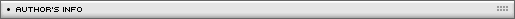Name: Zeh Fernando Location: São Paulo, SP, Brazil Age: 26 Flash experience: since Flash 2, I like to believe I'm advanced user although I still have a lot to learn on the OOP field Job: Multimedia designer Website: http://www.fatorcaos.com.br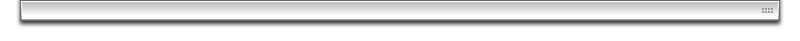| Homepage | News | Games | Articles | Multiplayer Central | Reviews | Spotlight | Forums | Info | Links | Contact us | Advertise | Credits || www.smartfoxserver.com | www.gotoandplay.biz | www.openspace-engine.com | gotoAndPlay() v 3.0.0 -- (c)2003-2008 gotoAndPlay() Team -- P.IVA 03121770048